## TN State Board 11th Chemistry Important Questions Chapter 9 Solutions

Question 1.
Define the following:

(i) Solution.
A solution is a homogeneous mixture of a solute and a solvent, where the solute is uniformly distributed throughout the solution.

(ii) Saturated solution.
A saturated solution more amount of the solute, than it can possibly dissolve at a particular temperature. Further, addition of the solute results in the precipitation of the solute.

(iii) Unsaturatgd solution.
An unsaturated solution is one which could dissolve some more quantity of the olute in a given amount of solvent, till it becomes saturated.

(iv) Super saturated solution.
A super saturated solution is one which contains more solute than the one require to form a saturated solution.Question 2.
Give examples for a homogeneous solution.
(i) Sea water
(ii) Air
(iii) Alloys like brass etc.

Question 3.
What do you understand by the term homogeneity?
The term homogeneity means that all the constituents of a solution are uniformly distributed throughout the solution.

Question 4.
Bring out the distinction between an aqueous and non aqueous solution.
An aqueous solution is one in which water is the solvent. Solution in which benzene, carbon tetrachloride, ether etc., used as solvents are known as non-aqueous solution.Question 5.
Give examples for:

(i) A gaseous solution.
Air (A mixture of nitrogen, oxygen and other gases). Humid oxygen (oxygen containing water). Camphor in nitrogen gas.

(ii) Liquid solution.
CO2 dissolved in water (carbonated water). Ethanol dissolved in water, salt water.

(iii) Solid solution.
Solution of H2 in palladium. Amalgam of potassium (used for dental filling). Gold alloy (of copper used in making jewellery).

Question 6.
What do you understand by the term

(i) Standard solution.
A standard solution or a stock solution is a Solution whose concentration is accurately known.

(ii) Working standard.
At the time of experiment, the solution , with required concentration is prepared by diluting the stock solution. This diluted solution is usually called working 1 standard.Question 7.
Describe how do you prepare

(i) A standard solution.
The required amount of the solute is weighed exactly in a weighing bottle. It is then transferred to a standard flask of definite volume. A small amount of water is added to dissolve the solute. Then water is added slowly till the mark indicated at the top of the flask. The flask is then covered with the stopper and the solution is shaken for uniform concentration.

(ii) Working standard.
A standard solution can also be called a stock solution, eg: If a 1M solution of a solute is prepared (1 mole of a solute present in 1 litre). A small known amount of the solution is taken and further diluted to get a solution of known concentration.

This diluted solution, then can be used for experimental purposes. The concentration of the diluted solution is known and hence this is also a standard solution. This diluted solution is known as working standard.

The volume of the stock solution of known concentration, to be taken and how much 7 volume of water to be added to get the concentration of the working standard can be obtained by the relation.

CsVs = CwVw
Cs and Vs are the concentration and volume of the stock solution and Cw and Vw are the concentration and volume of the working standard.Question 8.
What are the advantages of using standard solutions?
(i) The error due to weighing the solute can be minimised by using concentrated stock solution that requires large quantity of solute.
(ii) We can prepare working standards of different concentrations by diluting the stock solution, which is more efficient Since consistency is maintained.
(iii) Some of the concentrated solutions are more stable and are less likely to support microbial growth than working standards used in the experiments.

Question 9.
Define the term solubility of a solute in a given solvent.
Solubility of a solute is the maximum amount of solute that can be dissolved in a specific amount of solvent at a specified temperature. The solubility of a substance at a given temperature is defined as the amount of the solute that can be dissolved in 100 g of the solvent at a given temperature to form a saturated solution.

Question 10.
Mention the factors influencing the solubility.
(i) The solubility of a solute in a given solvent depends on the nature of the solute and solvent. Generally ionic compounds are soluble in polar solvents like water and insoluble in non-polar solvents like benzene or toluene. Similarly covalent compounds are soluble in non-polar solvents and insoluble in polar solvents.
(ii) The solubility of a solid solute in a liquid increases with the increase in temperature.
(iii) If heat is liberated during the dissolution, the solubility of the solute decreases with increase in temperature. (For endothermic dissolution). If the dissociation is exothermic, the solubility increases with increase in temperature.
(iv) For a gaseous solute, the solubility . increases with the pressure of the gas , according to Henry’s law.Question 11.
What are solubility curves? What information do we get from such curves?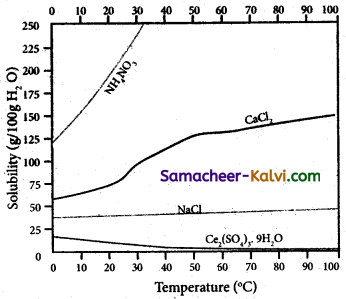The variation of solubility of a solid in given solvent at a given temperature i.e., plot of solubility curves temperature is known as solubility curves.
The information obtained from the solubility curves are:
(i) The solubility of sodium chloride does not vary appreciably as the maximum solubility is achieved at normal temperature.
; (ii) The dissolution process of ammonium nitrate is endothermic, the solubility increases steeply with increase in temperature.
(iii) In the case of ceric sulphate, the dissolution is exothermic and the solubility decreases with increase in temperature.
(iv) Even though the dissolution of calcium chloride is exothermic, the solubility increases moderately with increase in temperature. Here, the entropy factor also plays a significant role in deciding the position of the equilibrium.

Question 12.
Explain why the solubility of a gaseous solute in a liquid solvent decreases with increase in temperature.
The dissolution of gases in a liquid solvent is exothermic. i.e„ heat is liberated during the dissolution process. According to Eechatelier principle, increase in temperature, decreases the solubility of gas in a given solvent.

Question 13.
Why the carbonated drinks are stored in a pressurised container?
According to Henry’s law, the solubility of a gas increases with the pressure of the gas at a given temperature. Thus, to increase the solubility of CO2 in water, at the room temperature, CO2 gas is dissolved in water at high pressures.Question 14.
Compare Raoult’s Law and Henry ’s law.
Similarities:
(i) Both apply to the volatile component of the solution.
(ii) Both state that the vapour pressure of any component in solution is proportional to the mole fraction of the solute in solution.
pA = p°A XA (Raoult’s law)
PA = kH XA (Henry,s law)
where pA is the partial vapour pressure of the component A and XA is the mole fraction of the solute in solution.

Dissimilarities:
(i) The two laws in their proportionality constant. In Raoult’s law, it is p°A, the vapour pressure of pure component A and in Henry’s law it is Henry’s law constant, (kH). The value of kH is experimentally determined.
(ii) For every dilute solutions, solvent obeys. Raoult’s law while the solute obey Henry’s law.Question 15.
What are ideal and non-ideal solutions?
(i) Ideal solutions:
An ideal solution is one in which each component i.e., the solute as well as the solvent obeys the Raoult’s law over the entire range of concentration.
(ii) Non-ideal solutions:
The solutions which do not obey Raoult’s law over the entire range of concentration are called non-ideal Solutions.

Question 16.
Bring out the characteristics of ideal and non-ideal solutions.
Characteristics of ideal solutions:
(i) There is no change in the volume on . mixing the two components (solute & solvents). (∆Vmixing = 0).
(ii) There is no exchange of heat when the solute is dissolved in solvent (∆Hmixing = 0).
(iii) Escaping tendency of the solute and the solvent present in it should be same as in pure liquids.

Characteristics of non-ideal solutions:
For a non-ideal solution, there is a change in the volume and enthalpy upon mixing, i.e., ∆Hmixing ≠ 0 and ∆Vmixing ≠ 0. The deviation of the non-ideal solutions from the Raoult’s law can either be positive or negative.Question 17.
What type of deviation from ideal behaviour exhibited by a mixture of ethanol and water? Explain.
(i) A mixture of ethanol and water exhibit positive deviation from ideal behaviour. Hydrogen bonding exists between ethanol- ethanol, water-water and ethanol-water molecules as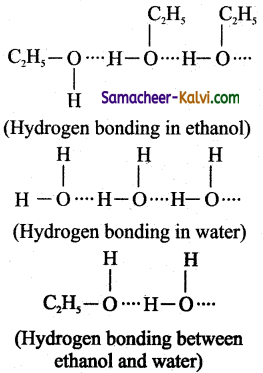The strength of hydrogen bonding between ethanol and water is less compared to thatof ethanol-ethanol or water-water. As a result, the escaping tendency of ethanol / water molecule is higher and hence both readily get evoporated. Thus, the partial vapour pressure of ethanol or water, pA or pB is greater then partial vapour, if this mixture behaves ideally.’ Hence, this – mixture shows positive deviation from ideal behaviour.

(ii) The volume of the solution is greater than the volume of ethanol and water taken together i.e., ∆Vmixing > 0.
It is due to the intermolecular forces among the molecules in solution. The molecules are loosely held, which results in an increase in volume on mixing.

(iii) ∆H(mixing) > 0. i.e., Heat is absorbed when ethanol and water are mixed. It is due to the fact that energy is required to break the hydrogen bond that exists between ethanol. and water molecules. This energy is less than the energy released in the formation of ethanol-water hydrogen bond.Question 18.
Give examples for non-ideal solution sharing positive deviation from ideal behaviour.
Ethyl alcohol and cyclohexane, Benzene and acetone, Carbon tetrachloride and chloroform, Acetone and ethyl alcohol, Ethyl alcohol and water.

Question 19.
What type of deviation does a mixture of aniline and phenol show? Explain.
(i) A liquid mixture of aniline and phenol exhibit negative deviation from ideal behaviour. In this mixture, there exists aniline-aniline, phenol-phenol and phenol-aniline interactions. These infractions are due to hydrogen bonding among themselves. The strength of the hydrogen, bond is greater in phenol and aniline, compared to phenol – phenol or aniline- aniline molecules.

The formation of hydrogen bond between phenol and aniline, increases the escaping tendency of both phenol and aniline. Hence, the experimentally observed vapour pressure of die solution is greater than the calculated vapour pressure based on Raoult’s law.

(ii) The total volume of the solution is less the volumes of phenol and aniline that is mixed. ∆Vmixing < 0.

(iii) There is an increase in the enthalpy of mixing, i.e., ∆Hmixing > 0. It is due to the fact that less energy is required to break phenol-phenol and aniline- required to form new hydrogen bonding between phenol and aniline.Question 20.
Give examples of a liquid mixtures showing negative deviation from ideal behaviour.
Acetone + chloroform, Chloroform + diethyl ether, Acetone + aniline, Chloroform + Benzene.

Question 21.
Mention the factors responsible for deviation from Raoult’s Law.
(i) Solute-solvent interaction: If solute- solute, solvent-solvent interactions are different from solute – solvent interactions, the liquid mixture deviate from ideal behaviour.
(ii) If the solute undergoes association or dissociation m a given solvent, then the solution deviate from ideal behaviour.
(iii) At higher temperatures, solution deviate from ideal behaviour.
(iv) The solution deviates from ideal behaviour at higher pressure.
(v) At relatively higher concentrations of the solutions; the deviations become larger.

Question 22.
Define Colligative properties.
The properties which do not depend on the chemical nature of the solute but depends only on the number of solute particles (ions/ molecules) present in the solution are known as colligative properties.Question 23.
Explain why the vapour pressure of a pure solvent is decreased by the addition of a non-volatile solid?
Addition of a non-volatile, non-electrolyte solute decreases the vapour pressure of the pure solvent. In other words, the vapour pressure of the solution is less than that of the – pure solvent. When the liquid and its vapour are in equilibrium, the standard free energy change, ∆G = 0. During the dissociation the free energy decreases due to increase in entropy, i.e.,

∆G = Gsolution – Gsolvent
In order to maintain equilibrium, the free energy of the vapour phase of the solvent must also decrease. The free energy of the vapour must also decrease to reduce the pressure. Thus the vapour pressure of the solution is less than that of the pure solvent.

(Alternatively)

Addition of a non-volatile, non-electrolyte solute to a pure solvent, decreases the number of solvent molecules on the surface of the solution. This decreases the escaping tendency of the solvent molecules in the surface of the solution. In other words, the vapour pressure solution is less than that of the pure solvent.

Question 24.
What do you understand by the term ‘relative lowering of vapour pressure’? Explain why it is called colligative property.
The ratio between the lowering of vapour pressure to the vapour pressure of pure solvent is known as relative lowering of vapour pressure i.e.,
$$\frac{\mathrm{p}^{\circ}-\mathrm{p}}{\mathrm{p}^{0}}$$ = Relative lowering of vapour pressure.

From the equation,

$$\frac{p_{(\text {solvent })}^{0}-\mathbf{p}_{(\text {solution })}}{\mathbf{p}_{\text {(solvent) }}^{0}}$$ = XB (mole fraction of the solute)

the relative lowering of vapour pressure is independent of its nature and depends only on the mole fraction of the solute. Hence, it is a colligative property.Question 25.
Derive the relationship between the relative lowering of vapour pressure and molecular weight of a non-volatile solute.
According to Raoult’s law the relative lowering of vapour pressure is,
$$\frac{\mathrm{P}^{0}{ }_{\text {solvent }}-\mathrm{P}_{\text {solution }}}{\mathrm{P}^{\mathrm{O}}}_{\text {solvent }}$$ = XB

Let wA and wB be the weights of the solvent and solute respectively and their corresponding molar masses are MA and MB, then the mole fraction of the solute XB is

XB = $$\frac{n_{\mathrm{B}}}{n_{\mathrm{A}}+n_{\mathrm{B}}}$$

Here, nA and nB are the moles of the solvent and the solute respectively. For dilute solutions nA >> nB. Hence nA + nB ≈ nA
Now,

XB = $$\frac{n_{\mathrm{B}}}{n_{\mathrm{A}}}$$

Number of moles of solvent and the solute are,

nA = $$\frac{w_{\mathrm{A}}}{\mathrm{M}_{\mathrm{A}}}$$,

nB = $$\frac{w_{\mathrm{B}}}{\mathrm{M}_{\mathrm{B}}}$$

Therefore,

XB = $$\frac{\frac{w_{\mathrm{B}}}{\mathrm{M}_{\mathrm{B}}}}{\frac{w_{\mathrm{A}}}{\mathrm{M}_{\mathrm{A}}}}$$

Thus, Relative lowering of vapour pressure = $$\frac{\frac{w_{\mathrm{B}}}{\mathrm{M}_{\mathrm{B}}}}{\frac{w_{\mathrm{A}}}{\mathrm{M}_{\mathrm{A}}}}$$

Relative lowering of vapour pressure = $$\frac{w_{\mathrm{B}} \times \mathrm{M}_{\mathrm{A}}}{w_{\mathrm{A}} \times \mathrm{M}_{\mathrm{B}}}$$

From the equation the molar mass of the solute (MB) can be calculated using the known values of wA, wB, MA and the measured relative lowering of vapour pressure.Question 26.
Define boiling point of a liquid.
The boiling point of a liquid is the temperature at which its vapour pressure becomes equal to the atmospheric pressure (1 atm).

Question 27.
Define elevation in boiling point.
The increase in boiling point produced when a non-volatile solute is added to a volatile solvent is known as the elevation in boiling point.

Question 28.
Explain why the boiling point of a solution of a non-volatile solute is higher than the boiling point of the pure solvent.
Addition of a non-volatile solute lowers the vapour pressure of the solvent, i.e., the solution has a lower vapour pressure than the solvent. Boiling point is the temperature at which vapour pressure of the liquid becomes equal to atmospheric pressure.

Since the vapour pressure of the solution is less than the vapour pressure of file solvent, its temperature has to be raised, more than that of the pure solvent So that its vapour pressure becomes equal to atmospheric pressure.Question 29.
How is elevation in boiling point related to the molecular weight of the solute?
The elevation of boiling point is directly proportional to the concentration of the solute particles.
∆Tb ∝ m ………..(1)
m is the concentration of solution expressed in molality.
∆Tb = Kb m
Where Kb</sub = molal boiling point elevation constant or Ebullioscopic constant.

Tb = Kb × $$\frac{w_{\mathrm{B}}}{\mathrm{M}_{\mathrm{B}}} \times \frac{1000}{w_{\mathrm{A}}}$$

Where wA, wB are the weights of solvent and solute respectively. MB is the molar mass solute B.

Question 30.
Define molal elevation constant.
The Kb molal elevation constant is equal to the elevation in boiling point for 1 molal solution.Question 31.
Define freezing point of a liquid.
Freezing point is defined as “the temperature at which the solid and the liquid states of the substance have the same vapour pressure”. At freezing point, the solid and liquid phases of the substance are in equilibrium.

Question 32.
Define depression in freezing point.
The lowering of the freezing point of the solvent when a solute is added is called depression in freezing point (∆Tf).

Question 33.
Explain why freezing point of a solution (T) is lower than that of the freezing point of pure solvent (T)?
Curve ABC gives the variation of the vapour pressure of the pure solvent with temperautre. At B, the liquid is in equilirbium with the solid solvent, (in the case of H2O, the equilibrium is ice (s) ⇌ water (l)). The temperature is 0°C and the pressure is 1 atm.

At this temperature, the vapour pressure of liquid and that of solid solvent are equal. This is known as the freezing point of the pure solvent (T°f)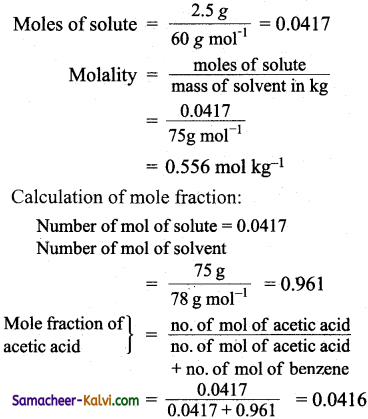Addition of non-volatile, non-electrolyte solute to the pure solvent decreases the vapour pressure of solvent to D. i.e., the vapour pressure of the solution is indicated by the point D. Decrease in temperature decreases the vapour pressure of the solution.

At ‘E’, i. e., the freezing point of the pure solvent, the solution does not freeze. This is because, the vapour pressure of the solution is not equal to vapour pressure of the pure solvent. The temperature has to be decreased to the point ‘F’, where the vapour pressure of the solution . and the pure solvent becomes equal, i.e., the freezing point of the solution is less than the freezing point of the pure solvent.Question 34.
How is depression in freezing point related to the molar mass of the non-volatile solute?
The depression in freezing point is directly proportional to the molal concentration of the solute particles.
Hence,
∆Tf ∝ m
∆Tf = Kf m
Here, ‘m’ = is the molality of the solution.

∆Tf = Kf × $$\frac{w_{\mathrm{B}}}{\mathrm{M}_{\mathrm{B}}} \times \frac{1000}{w_{\mathrm{A}}}$$

Where m = $$\frac{w_{\mathrm{B}}}{\mathrm{M}_{\mathrm{B}}} \times \frac{1000}{w_{\mathrm{A}}}$$

wA and wB are the weights of solvent (A) and solute (B) respectively. MB is the gram molecular weight of the solute and Kf is molal depression constant.

Question 35.
What is a semipermeable membrane?
The semipermeable membrane is oneAn which selectively allows certain molecules in the solution to pass through it but not others.Question 36.
Define osmotic pressure.
Osmotic pressure can be defined as “the pressure that must be applied to the solution to stop the influx of the solvent (to stop osmosis) through the semipermeable membrane”.

Question 37.
Write van’t Hoff equation to calculate osmotic pressure.
π = cRT .
Here, c = Concentration of the solution in molarity
T = Temperature
R = Gas constant.

Question 38.
Derive an equation for determination of molar mass from osmotic pressure.
According to Van’t Hoff equation
π = cRT
c = $$\frac{n}{\mathrm{~V}}$$
Here, n = number of moles of solute dissolved in ‘V’ litre of the solution.
Therefore, n = $$\frac{n}{\mathrm{~V}}$$RT or
πV = nRT ………..(1)

If the solution is prepared by dissolving wB g of non-volatile solute in wA g of solvent, then the number of moles ‘n’ is,
n = wB / MB
Since, MB = molar mass of the solute Substituting the V in (1), we get,

π = $$\frac{w_{\mathrm{B}}}{\mathrm{V}} \frac{\mathrm{RT}}{\mathrm{M}_{\mathrm{B}}}$$

MB = $$\frac{w_{\mathrm{B}}}{\mathrm{V}} \frac{\mathrm{RT}}{\pi}$$ ………(2)
From the equation (2), molar mass of the solute can be calculated.Question 39.
Explain why osmotic pressure measurements are prepared to determine the molar mass of polymers than other colligative properties.
Unlike elevation of boiling point (for 1 molal solution the elevation in boiling point is 0.512°C for water) and the depression in freezing point (for 1 molal solution the depression in freezing point is 1.86°C for water), the magnitude of osmotic pressure is large.

The osmotic pressure can be measured at room temperature enables to determine the molecular mass of biomolecules which are unstable at higher temperatures.

Even for a very dilute solution, the osmotic pressure is large.

Question 40.
What are ‘hypotonic’ and ‘hypertonic’ solutions?
These terms are used to compare the relative osmotic pressure of two solutions. A solution of low osmotic pressure is termed hypotonic and the one with higher osmotic pressure is terms as hypertonic.

Question 41.
Explain the principle involved in intravenous drip of saline water,
Intravenous drip of saline water: Saline drip to the patients is based on the principle of osmosis.
(i) A 0.91% solution of NaCl in water is isotonic with blood. Hence, in this solutions red blood cells would neither
swell or shrink.
(ii) Aqueous solution of NaCl with concentration less than 0.91 % is hypotonic to blood. On placing red blood cells in this solution the solvent from outside of the cells will flow into the cell to normalise the osmotic pressure. This process is called hemolysis, which causes the cells to burst.
(iii) Aqueous solution ofNaCl with concentration more than 0.91% is hypertonic to blood. On placing red blood cells in this solution, the solvent molecules flow out of the cell which causes the cell to shrink.Question 42.
Define reverse osmosis.
It can be defined as a process in which a solvent passes through a Semipermeable membrane in the opposite direction of osmosis, when subjected to a hydrostatic pressure greater than the osmotic pressure.

Question 43.
Mention the applications of reverse osmosis.
Application of reverse osmosis:
(i) Used in desalination of sea water and also in the purification of water.
(ii) To prepare fruit juice concentrate.
(iii) To remove pollutants from sewage disposal.

Question 44.
What do you understand by ‘abnormal molar mass’?
The molar masses of solutes which undergo dissociation or association in a given solvent, if determined by measuring any of the colligative properties iS found to be different from their actual value. This is known as abnormal molar mass.Question 45.
Define Van’t Hoff factor.
The extent of dissociation or association of a solute in a given solvent is given by Van’t Hoff factor (i). It is defined as the ratio of the actual molar mass to the abriormal (calculated) molar mass of the solute. Here, the abnormal molar mass is the molar mass calculated usingthe experimentally determined colligative property.
i = $$\frac{\text { Normal (actual) molar mass }}{\text { Observed (abnormal) molar mass }}$$

= $$\frac{\text { Observed colligative property }}{\text { Calculated colligative property }}$$

Question 46.
How do you determine the nature of solute from Van’t Hoff factor?
(i) If i = 1, the solute neither undergoes dissociation or association in a given solvent. The observed molar mass and the normal mass will be the same.
(ii) If i> 1, the solute undergoes dissociation in a given solvent. The observed molar mass will be less than the normal molar mass.
(iii) If i < 1, the solute undergoes association in a given solvent. The observed molar mass will be greater than the normal molar mass.Question 47.
Calculate the mole fraction and molality of 2.5 gram of ethanoic acid (CH3COOH) in 75 gram of benzene.Question 48.
Calculate the molarity and normality of a solution containing 5,gram of NaOH in 450 ml solution.
Mass of NaOH dissolved = 5 g
Volume of the solution = 450 cm3
Molar mass of NaOH = 40 g mol-1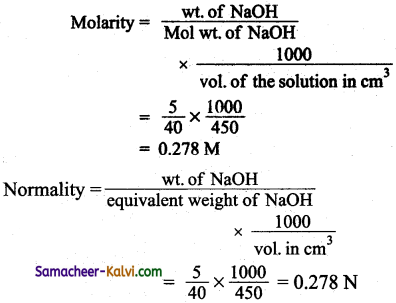(Normality and molarity of NaOH are the same. NaOH is monoacidic base).Question 49.
20 ml of 10 N HCl are diluted with distilled water to form one litre solution. What is the normality of the diluted solution?
During dilution, the number of equivalents of the solute remains the same, but the concentration (normality) decreases.
No. of equivalents (Before dilution) = No. of equivalents (after dilution)
V1 N1 x 10-3 = V2 N2 x 10-3
V1 = 20 ml; V2 = 1000 ml
N1 = 10 N ; N2 = ?
20 × 10 = 1000 × N2 20 × 10
N2 = $$\frac{20 \times 10}{1000}$$ = 0.2 N

Question 50.
If N2 gas is bubbled through water at 293 K, how many millimoles of N2 gas would dissolve in 1 litre of water. Assume N2 exerts a partial pressure of 0.987 bar. Given that Heniy’s law constant for N2 at 293 K is 76.48 bar.
According to Henry’s law.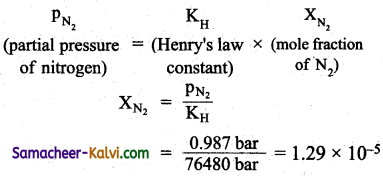If V moles of N2 are present in 1 litre of H2O (i.e., 55.5 moles),
XN2 = $$\frac{n}{n+55.5}$$

= $$\frac{n}{55.5}$$ (n <<55.5)
∴ $$\frac{n}{55.5}$$ = 1.29 × 10-5
n = 1.29 × 10-5 × 55.5
= 71.595 × 10-5 moles
= 0.716 millimetersQuestion 51.
Vapour pressure of chloroform (CHCl3) and dichloromethane (CH2Cl2) at 298 K are 200 mm Hg and 415 mm Hg respectively. Calculate:
(i) Vapour pressure of the solution prepared by mixing 25.5 grams of CHCl3 and 40 g of CH3Cl2 at 298 K.
Calculation of vapour pressure of the solution:
mass of CHCl3 = 25.5 g
mass of CH2Cl2 = 40 g
molar mass of CHCl3 = 12 + 1 + 3 × 35.5 = 119.5 g mol-1
molar mass of CH2Cl2 = 12 + 2 + 2 × 35.5 = 85 g mol-1
∴ moles of CHCl3 = $$\frac{25.5 g}{119.5 g \mathrm{~mol}^{-1}}$$
= 0.213 mol

∴ moles of CH2Cl2 = $$\frac{40 g}{85 g m o l^{-1}}$$ = 0.470 mol

∴ mole fraction of CHCl3 (XCHCl3) = $$\frac{0.213}{0.213+0.470}$$ = 0.312

mole fraction of CH2Cl2(XCH2Cl2) = 1 – 0.32 = 0.688

PCHCl3 = P°CHCl3 × XCHCl3
= 200 × 0.312

PCH2Cl2 = P°CH2Cl2 × XCH2Cl2

= 415 × 0.688

Ptotal = PCHCl3 PCH2Cl2
= 200 × 0.312 + 415 × 0.688 = 347.9 mm

(ii) The mole fraction of each component in the vapour phase.
Calculation of mole fraction of each component in vapour phase.
As calculated above,
PCHCl3 = 62
PCl3Cl2 = 285.5
Ptotal = 347.9
Mole fraction of CHCl3 in the vapour phase = $$\frac{\mathrm{p}_{\mathrm{CHCl}_{3}}}{\mathrm{P}_{\text {total }}}$$

= $$\frac{62.4 \mathrm{~mm}}{347.9 \mathrm{~mm}}$$ = 0.18

Mole fraction of CH3Cl2 in the vapour phase = 1 – 0.18 = 0.82Question 52.
Vapour pressure of water at 293 K is 17.51 mm. Lowering of vapour pressure if a sugar solution is 0.0614 mm. Calculate
(i) Relative lowering of vapour pressure.
Given:
Vapour pressure of H2O (p°) =17.511 mm
Lowering of vapour pressure (p° – p) = 0.0614 mm
Relative lowering of vapour pressure = $$\frac{\left(p^{o}-p\right)}{p^{0}}$$

= $$\frac{0.0614 \mathrm{~mm}}{17.51 \mathrm{~m}}$$ =000351

(ii) Vapour pressure of the solution.
Vapour pressure of the solution (p)
p° – p = 0.0614
(17.51 mm – p) = 0.0614 mm
p = 17.51 mm-0.0614 mm = 17.4468 mm

(iii) Mole fraction of water.
To calculate the mole fraction of water.
By Raoult’s law $$\frac{\left(p^{0}-p\right)}{p^{0}}$$ = X2 (mole fraction of solute)
X2 = 0.00351
mole fraction of water = 1 – 0.00351 = 0.99649Question 53.
The vapour pressure of a 5 % solution of a non volatile organic substance at 373 K is 745 mm. Calculate the molar mass of the solute.
Mass of the solute (w2) = 5 g
Mass of the solution = 100 g
Mass of the solvent (w1) = 100 – 5 = 95 g
Vapour pressure of pure water at 373 K (p°) = 760 mm
Vapour pressure of the solution at 373 K (p) = 745 mm (given)
Molar mass of solvent (M1) = 18 g mol-1
Molar mass of solute = ?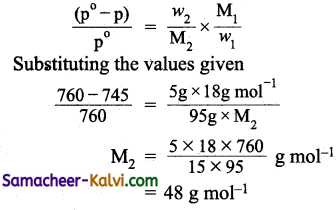Question 54.
200 cm3 ofan aqueous solution of protein contains 1.26 g of protein. The osmotic pressure of the solution at 300 K is found to be 2.57 × 10-3 bar. Calculate the molar mass of protein.
Given:
Mass of the protein (w2) = 1.26 g
Volume of the solution (v) = 200 cm3 = 0.200 L
Osmotic pressure (π) = 2.57 × 10-3 bar
Temperature (T) = 300 K
R = gas constant = 0.083 L bar K-1 mol-1
M2 = $$\frac{w_{2} \times R \times T}{\pi v}$$

= $$\frac{1.26 \mathrm{~g} \times 0.083 \mathrm{~L} \mathrm{bar} \mathrm{K}^{-1} \mathrm{~mol}^{-1} \times 300 \mathrm{~K}}{2.57 \times 10^{-3} \mathrm{bar} \times 0.200 \mathrm{~L}}$$

= 61039 g mol-1

Question 55.
The boiling point of benzene is 353.23 K. When 1.80 g of a non-volatile solute was dissolved in 90 g of benzene, the boiling point is raised to 354.11 K. Calculate the molar mass of the solute. K6 for benzene is 2.53 K kg mol-1.
Given:
Weight of the solute (w2) = 1.80 g
Weight of the solvent (w1) = 90 g
Boiling point of solution (T) = 354.11 K
Boiling point of the solvent (T0) =353.23 K
Molar elevation constant for benzene (Kb) = 2.53K kg mol
Molecular weight of the solute = ?
Using the formula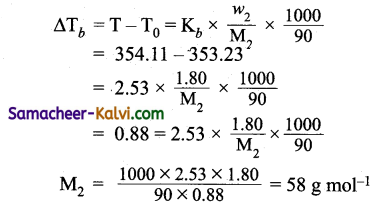Question 56.
45 g of ethelene glycol (C2H6O2) is mixed with 600 g of water. Calculate
(a) freezing point depression.
(b) freezing point of the solution.
(Kf for water = 1.86 K kg mol-1 Atomic mass of C = 12, H = 1; O = 16 amu).
Given:
Weight of the solute (w2) = 45 g
Weight of the solvent (w1) = 600 g
Molal depression constant (Kf) = 1.86 kg mol-1
Molar mass of solute (M2) = 24 + 6 + 32 = 62 g mol-1
Substituting these values in
∆Tf = Kf × $$\frac{w_{2}}{\mathrm{M}_{2}} \times \frac{1000}{w_{1}}$$

∆Tf = $$\begin{gathered} 1.86 \mathrm{~K} \mathrm{~kg} \mathrm{~mol}^{-1} \\ \frac{\times 45 \mathrm{~g} \times 1000}{62 \mathrm{~g} \mathrm{~mol}^{-1} \times 600 \mathrm{~g}} \end{gathered}$$

Freezing point of pure water = 273.15 K

Freezing point of the solution = 273.15 – 2.25 = 270.9 KQuestion 57.
0.5 g KCl was. dissolved in 100 g of water and the solution originally at 20°C froze at – 0.24°C. Calculate the percent ionisation of the salt.
Kf for water per 1000 g water = 1.86°C
Weight of KCl3 (w2) = 0.5 g
Weight of water (w1) = 100 g
Molecular weight of KCl (m2) = 39 + 35.5 = 74.5 g
∆Tf = 0 – (-0.24)
Van’t Hoff factor (i) for KCl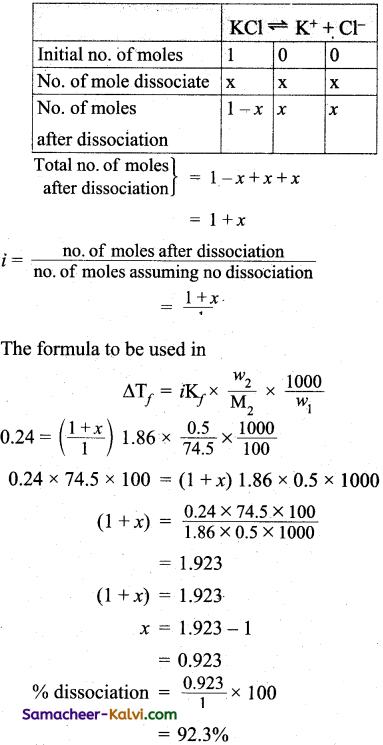Question 1.
The normality of 0.1M H2S04 is equal to:
(a) 0.2 N
(b) 0.1 N
(c) 0.05 N
(d) 2.0 N
(a) 0.2 N
Hint:
Normality of a solution of acid = molarity × basicity
N = 0.1 × 2 (H2SO4 is dibasic) = 0.2 N

Question 2.
Which unit of concentration value is not changed with the change in temperature?
(a) Normality
(b) Molarity
(c) %V / V
(d) molality
(d) molality
Hint:
m = $$\frac{\text { no. of mol of solute }}{\text { mass of solvent in } \mathrm{Kg}}$$

The masses of the solute and the solvent has no influence on temperature.Question 3.
On increasing the temperature the solubility of gaseous solute in liquid solvent:
(a) increases
(b) decreases
(c) remains constant
(d) uncertain
(b) decreases
Hint:
Dissolution of a gas in a liquid is an endothermic process. According to Le-Chatelier’s principle, increase in temperature result in decrease in the solubility of a gas.

Question 4.
What is the molality for 10% of w/w NaOH (aqueous) solution?
(a) 2.778
(b) 2.5
(c) 10
(d) 5
(a) 2.778
Hint:
Weight of the solution = 100 g
Weight of NaOH = 10 g
Weight of water = 90 g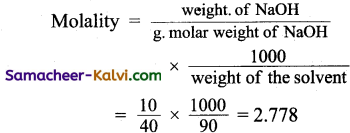Question 5.
0.05 M glucose solution is isotonic with which of the following solution?
(a) 0.10 M NaCl
(b) 0.05 M NaCl
(c) 0.025 M NaCl
(d) 1 M NaCl
(c) 0.025 M NaCl
Hint:
π ∝ C glucose contains only glucose molecules and NaCl contains 2 particles (viz Na+ + Cl). Isotonic solution should have same molar concentration.
0.025 M NaCl = 0.05 NaCl .
0.01 M NaCl = 0.02 M NaCl
0.05 M NaCl = 0.1 M NaCl
1 M NaCl = 2 M NaClQuestion 6.
Two solutions having same osmotic pressure at a given temperature are called:
(a) hypertonic
(b) hypotonic
(c) isotonic
(d) supertonic
(c) isotonic

Question 7.
Van’t Hoff factor are l and m in the case of substances undergoing association, dissociation respectively. Then their Van’t Hoff factor will be:
(a) l < m
(b) l = m
(c) m < l
(d) l = m = 1
(a) l < m
Hint:
Van’t Hoff factor l < 1 for solutes undergoes association and l > 1 for solutes undergoing dissociation.
∴ l < m

Question 8.
The Van’t Hoff factor for which of the following solute will be greater than 1:
(a) glucose
(b) benzoic acid
(c) sodium sulphate
(d) sucrose
(c) sodium sulphate
Hint:
Na2SO4 → 2 Na+ + SO4-2
Glucose and sucrose does not undergo association or dissociation. Whereas, benzoic acid undergoes association.
Van’t Hoff factor is > 1 for solutes which undergo dissociation in a given solvent.Question 9.
Which one of the following will give a better result when used on the roads to avoid freezing in the cold countries.
(a) NaCl
(b) CaCl2
(c) Na2SO4
(d) AlCl3
(d) AlCl3
Hint:
Greater the number of ions produced greater is the depression in freezing point. In cold countries, salts are added to prevent freezing of water (forfnation of ice).

Question 10.
The lowest freezing point of 0.1 M aqueous solution is for:
(a) K2SO4
(b) NaCl
(c) Urea
(d) Glucose
Hint: Greater the number of particles, greater is the depression in freezing point. Hence a solution which contains fnore number of ions will have the lowest freezing point K2SO4 gives three ions.

Question 11.
Out of the following which has the highest boiling point will be:
(a) 0.1 M NaCl
(b) 0.1M BaCl2
(c) 0.1M KNO3
(d) 0.1 M K4[Fe(CN)6]
(d) 0.1 M K4[Fe(CN)6]
Hint:
K4[Fe(CN)6] ionises to give 4K+ and one Fe(CN)6-4 ion. i.e., 5 ions. Greater the number of particles (ions) greater is the elevation in freezing point. Hence the solution which gives more number of ions will have the highest ‘ boiling point.Question 12.
Elevation in boiling point of a molar glucose solution (d = 1.2g mL-1):
(a) 0.98 Kb
(b) Kb
(c) 1.20Kb
(d) 1.02Kb
(a) 0.98 Kb
Hint:
1 mL of glucose solution = 1.2 g
1000 mL of glucose solution = 1.2 x 1000
Weight of the solvent = 1200 – 180 = 1020 g
w2 = m2 = 180;
∆Tb = Kb = $$\frac{w_{2}}{m_{2}} \times \frac{1000}{w_{1}}$$

= Kb × $$\frac{180}{180} \times \frac{1000}{1020}$$

= 0.98 Kb = 12000 g

Question 13.
The Henry’s law constant does not depend on the:
(a) nature of solute
(b) nature of solvent
(c) temperature of the system
(d) external pressure of the gas on the System
(b) nature of solvent
Hint:
Henry’s law constant depends on the nature of the gas, temperature and pressure of the gas.

Question 14.
The unit of molality is:
(a) mol
(b) mol dm-3
(c) mol kg-1
(d) mol-1
(c) mol kg-1Question 15.
What is the concentration of a solution prepared by diluting 0.5 L of 1.80 M to 1.5 L with solvent?
(a) 0.6 M
(b) 0.5 M
(c) 0.8 M
(d) 0.7 M
(a) 0.6 M
Hint:
During dilution, the number of mol of the solute does not change.
Hence, V1 M1 = V2 M2
Number of mole before dilution = Number of mol after dilution
5 L × 1.80 M = 1.5 L × M2
M2 = $$\frac{5 \mathrm{~L} \times 1.80 \mathrm{M}}{1.5 \mathrm{~L}}$$ = 0.6 M

Question 16.
What will be the concentration of sugar in a solution prepared by mixing 3.0 L of 2.0 M sugar with 2.5 L of 1.0 M Salt:
(a) 1.09 M
(b) 1.54 M
(c) 1.32 M
(d) 1.7 M
(a) 1.09 M
Hint:
The number of mol of sugar does not change on mixing but the volume of the solution change. The volume of the solution after mixing is
3.0 + 2.5 = 5.5 L
V1 M1 = V2 M2
3 L × 2.0 M = 5.5 L × M2
M2 = $$\frac{3 \mathrm{~L} \times 2.0 \mathrm{M}}{5.5 \mathrm{~L}}$$ = 1.09 M

Question 17.
Assertion:
Molarity of a solution in liquid state changes with temperature.
Reason :
The volume of the solution will change with temperature.
(a) Both assertion and reason are true and reason is the correct explanation of assertion.
(b) Both assertion and reason are true but reason is not the correct explanation for assertion.
(c) Assertion is true but reason is false.
(d) Both assertion and reason are false.
(a) Both assertion and reason are true and reason is the correct explanation of assertion.Question 18.
Assertion:
When, methyl alcohol is added to water, boiling point of water increases.
Reason :
When a volatile solute is added to a volatile, solvent, elevation in boiling point is observed.
(a) Both assertion and reason are true and reason is the correct explanation of assertion.
(b) Both assertion and reason are true but reason is not the correct explanation for assertion.
(c) Assertion is true but reason is false.
(d) Both assertion and reason are false.
(d) Both assertion and reason are false.
Hint:
When methyl alcohol is added to water, boiling point of water decreases (correct assertion). When a volatile solute is added to a volatile solvent, the vapour pressure of solvent increases.

Question 19.
Assertion:
When sodium chloride is added to water, a depression in freezing – point is observed.
Reason :
The lowering of vapour pressure of a solution, causes the depression in freezing point.
(a) Both assertion and reason are true and reason is the correct explanation of assertion.
(b) Both assertion and reason are true but reason is not the correct explanation for assertion.
(c) Assertion is true but reason is false.
(d) Both assertion and reason are false.

Question 20.
Assertion:
If 100 ml of 0.1 M solution of glucose has higher depression in freezing point than 100 ml of 0.1 M solution of urea.
Reason :
Kf for glucose and urea are different.
(a) Both assertion and reason are true and reason is the correct explanation of assertion.
(b) Both assertion and reason are true but reason is not the correct explanation of assertion.
(c) Assertion is true but reason is false
(d) Both assertion and reason are false.
(d) Both assertion and reason are false.
Hint:
Correct assertion: Depression in freezing point of 0.1 M solution of glucose and urea are the same.
Correct reason: Kf is a property of solvent.Question 21.
Assertion:
Out of various eolligative properties, osmotic pressure is used for the determination of various polymers.
Reason:
Polymer solutions do not possess constant boiling point or freezing i point.
(a) Both assertion and reason are true and reason is the correct explanation of assertion.
(b) Both assertion and reason are true but reason is not the correct explanation for assertion.
(c) Assertion is true but reason is false.
(d) Both assertion and reason are false.
(a) Both assertion and reason are true and reason is the correct explanation of assertion.

Question 22.
Solutions of different types are given below. Identify the solution which contain a different solvent from the rest.
(a) Hydrated salts
(b) Alloys
(c) Dissolved gas in a mineral
(d) Alcohol in water
(d) Alcohol in water
Hint:
Liquid is the solvent, while in all others the solvent is a solid.

Question 23.
Among the solids given, identify the one, which on dissolution absorbs heat.
(a) LiCO3
(b) Na2CO3.H2O
(c) Ce2(SO4)3
(d) NaCl
(d) NaCl
Hint:
The dissolution of NaCl in H2O is endothermic, while the rest are exothermic.Question 24.
Which one of the following pairs will not form an ideal solution?
(a) C6H6 and CCl4
(b) C6H6 and C6H3CH3
(c) C2H5Br and C2H5I
(d) (CH3)2CO and CCl4
(d) (CH3)2CO and CCl4
Hint:
Structurally similar liquid form ideal solution.

Question 25.
Which of the following is correct for asolution sharing positive deviation from Raoult’s law?
(a) ∆V +ve; ∆H = +ve
(b) ∆V = -ve; ∆H = -ve
(c) ∆V = +ve; ∆H -ve
(d) ∆V = -ve; ∆H = +ve
(a) ∆V +ve; ∆H = +ve

Question 26.
Assertion:
The vapour pressure of 0.1M sugar solution is more than that of 0.1M KCl solution.
Reason:
Lowering of vapour pressure is directly proportional to the number of species present in the solution.
(a) If both assertion and reason are true and reason is the correct explanation of the assertion.
(b) If both assertion arid reason are true but reason is not the correct explanation of the assertion.
(c) If assertion is correct but reason is incorrect.
(d) If assertion is incorrect but reason is correct.
(a) If both assertion and reason are true and reason is the correct explanation of the assertion.Question 27.
Assertion:
Out of the various collegative properties, Osmotic pressure is used for the determination of molecular masses of polymers.
Reason:
Polymer solutions do not possess a constant boiling point.
(a) If both assertion and reason are true and reason is the correct explanation of the assertion.
(b) If both assertion and reason are true but reason is not the correct explanation of the assertion.
(c) If assertion is correct but reason is incorrect.
(d) If assertion is incorrect but reason is correct.
(c) If assertion is correct but reason is incorrect.

Question 28.
Assertion:
One molar solution is always more concentrated than a one molal solution.
Reason :
The amount of solvent is 1 M and 1 M aqueous solution is not equal.
(a) If both assertion and reason are true and reason is the correct explanation of the assertion.
(b) If both assertion and reason are true but reason is not the correct explanation of the assertion. .
(c) If assertion is correct but reason is incorrect.
(d) If assertion is incorrect but reason is correct.
(d) If assertion is incorrect but reason is correct.

Question 29.
Assertion:
If more and more solute is added to a solvent, the freezing point of the solution keeps on becoming higher and higher.
Reason :
Presence of large amount of solute does not allow the solution to freeze.
(a) If both assertion and reason are true and reason is the correct explanation of the assertion.
(b) If both assertion and reason are true but reason is not the correct explanation of the assertion.
(c) If assertion is correct but reason is incorrect.
(d) If assertion is incorrect but reason is correct.
(d) If assertion is incorrect but reason is correct.Question 30.
Assertion:
If a liquid solute more volatile than the solvent is added to the solvent, the vapour pressure of the solution (ps) increases i.e.,ps > p.
Reason:
In the presence of a more volatile liquid solute, only the solute will form the vapours and solvent will not.
(a) If both assertion and reason are true and reason is the correct explanation of the assertion.
(b) If both assertion and reason are true but reason is not the correct explanation of the assertion.
(c) If assertion is correct but reason is incorrect.
(d) If assertion is incorrect but reason is correct.

Question 31.
Which of the following statement is incorrect?
(a) Greater the lowering of vapour pressure greater is the boiling point of the solution.
(b) Greater the lowering of vapour pressure, greater is the freezing point of the solution.
(c) At the freezing point, the solute and the solvent have che same vapour pressure.
(d) The units of molal depression constant are Km-1.
(b) Greater the lowering of vapour pressure, greater is the freezing point of the solution.
Hint:
Greater the lowering of vapour pressure, greater is the depression in freezing point i.e., lower is the actual freezing point.Question 32.
Which of the following is incorrect?
(a) Mass of the gas dissolved is directly proportional to its pressure.
(b) Mass of the gas dissolved is inversely proportional to temperature.
(c) A soda water bottle contains oxygen gas dissolved under pressure.
(d) Permanent gases are less soluble than temporary gases.
(c) A soda water bottle contains oxygen gas dissolved under pressure.
Hint:
A soda water bottle contains carbondioxide underpressure.

Question 33.
Choose the correct statement.
In the phenomenon of osmosis:
(a) Solvent molecules move from higher concentration to lower concentration.
(b) Solvent molecules move from lower concentration to higher concentration.
(c) Solute molecules move from higher concentration to lower concentration.
(d) Solute molecules move from lower concentration to higher concentration.
(b) Solvent molecules move from lower concentration to higher concentration.

Question 34.
Which of the following statement is correct?
(a) Lowering of vapour pressure takes place only in ideai solutions.
(b) Lowering of vapour pressure does not depend upon the solvent at a given concentration of the solute.
(c) Lowering of vapour pressure depends upon the nature of the solute.
(d) Relative lowering of vapour pressure does not depend upon the solvent at a given concentration of the solute.
(a) Lowering of vapour pressure takes place only in ideal solutions.Question 35.
For an ideal binary liquid solution with p°A > p°B, which of the following relations between xA(mole fraction of A in liquid phase) and yA(mole fraction of A in vapour phase) is correctly represented.
(a) xA = yA
(b) xA > yA
(c) xA < yA
(d) xA and yA cannot be correlated with each other.
(c) xA < yA
Hint:
As p°A > p°B, A is more volatile and hence yA > xA or xA < yA.

Question 36.
Match the entities of column I with appropriate entities of column II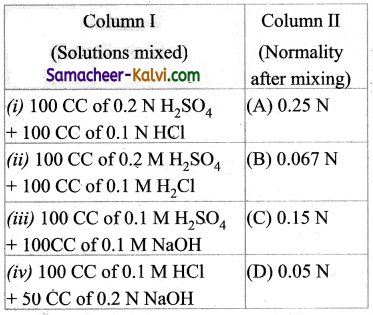(a) (i) – (C), (ii) – (D), (iii) – (A), (iv) – (B)
(b) (i) – (B), (ii) – (A), (iii) – (C), (iv) – (D)
(c) (i) – (C), (ii) – (A), (iii) – (D), (iv) – (B)
(d) (i) – (D), (ii) – (C), (iii) – (A), (iv) – (B)
(c) (i) – (C), (ii) – (A), (iii) – (D), (iv) – (B)Question 37.
Match the entities of column I with appropriate entities of column II.

 Column I (Vant Hoff factor i) Column II (Molecular state of the solute in a solution) (i) i > 1 (A) the solute under goes association (ii) i < 1 (B) the solute undergoes dissociation (iii) i = 1 (C) impossible (iv) i = 0 (D) the solute neither undergoes dissociation or association

(a) (i) – (A), (ii) – (B), (iii) – (D), (iv) – (C)
(b) (i) – (D), (ii) – (C), (iii) – (A), (iv) – (B)
(c) (i) – (C), (ii) – (D), (iii) – (B), (iv) – (A)
(d) (i) – (B), (ii) – (A), (iii) – (D), (iv) – (C)